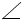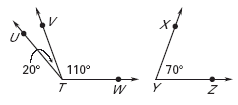# Complementary or Supplementary

To determine whether an angle pair is complementary or supplementary you have to recall the definition of complementary and supplementary.

Complementary - two angles whose sum is 90°

Supplementary - two angles whose sum is 180°

Example 1

Identify a pair of complementary and supplementary angles.

m1 = 40°

m2 = 140°

m3 = 50°

m1 + m3 = 90° therefore 1 and 3 are complementary.

m 1 + m 2 = 180° therefore 1 and 2 are supplementary.

Example 2: Determine if angles POQ and ROS are complementary or supplementary.mPOQ is 55°
mROS is 35°

55° + 35° = 90° Therefore the angles are complementary.

Example 3: Identify a pair of complementary and a pair of supplementary angles.mUTV is 20°

mVTW is 110°

mXYZ is 70°

mUTV + mXYZ = 20° + 70° = 90°UTV andXYZ are complementary

mVTW + mXYZ = 110° + 70° = 180°VTW andXYZ are supplementary

Example 4: Identify a pair of complementary and a pair of supplementary angles.•3 and4 form a right angle. A right angle is 90° therefore they are complementary.3 +4 = 90°

•1 and2 are a linear pair which means they are supplementary. m1 = 90° and m2 = 90°1 +2 = 90° + 90° = 180°

Quick Summary:

There are two methods to determining if an angle pair is complementary: (1) if you are looking at a diagram you will be looking for two adjacent angles that form a right angle.
(2) you can add the measure of any two angles and see if their sum is 90°.

There are two methods to determining if an angle pair is supplementary: (1) if you are looking at a diagram you will be looking for two adjacent angles that form a linear pair.
(2) you can add the measure of any two angles and see if their sum is 180°.

 Related Links: Math Geometry Topics Polygons Supplementary Angles

To link to this Complementary or Supplementary page, copy the following code to your site: